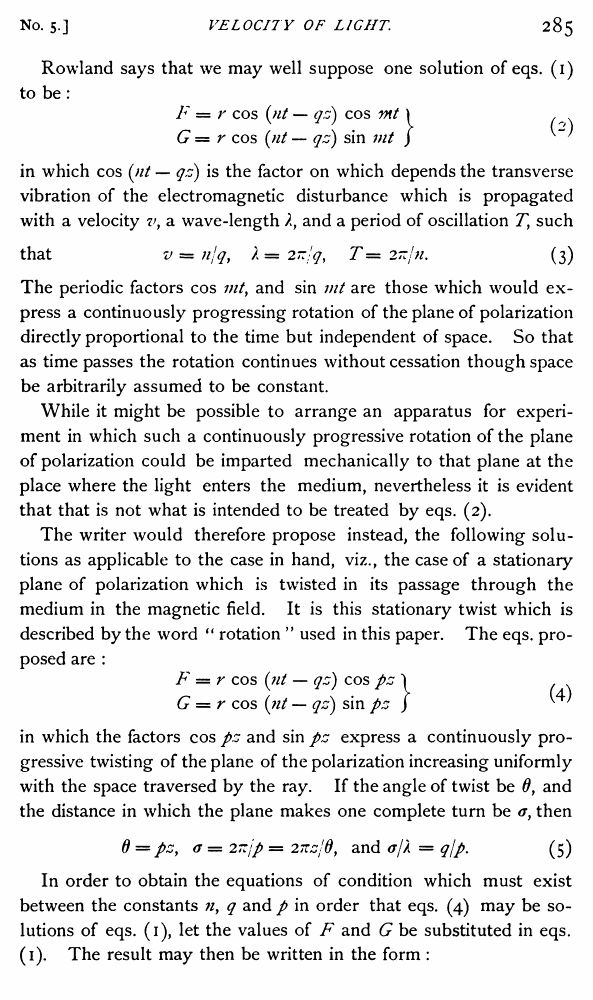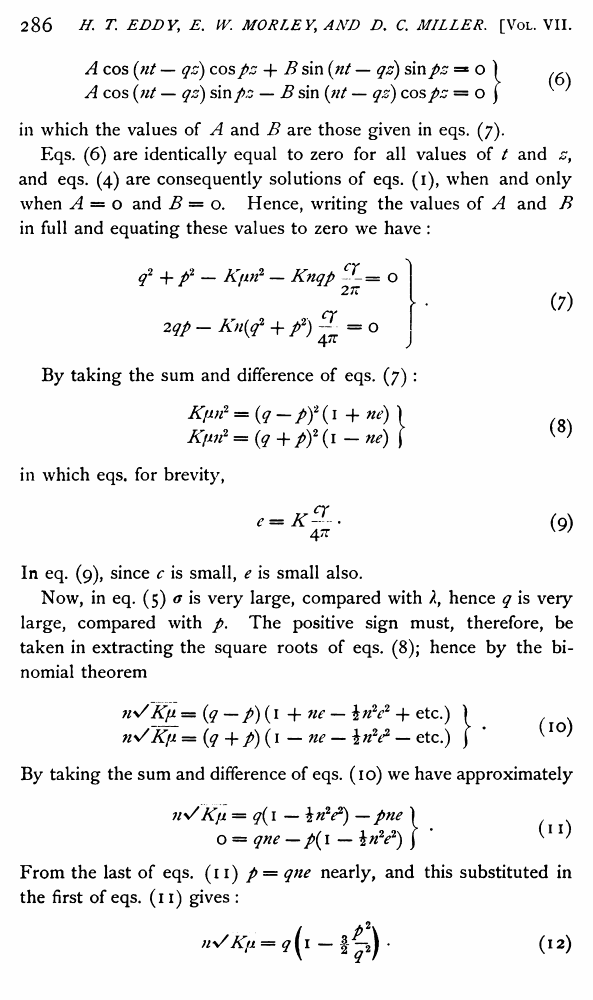Henry T. Eddy, Edward W. Morley, Dayton C. Miller. The Velocity of Light in The Magnetic Field //Physical Review (Series I), Volume 7, Issue 5 (1898)

В начало   Другие форматы   <<<     Страница 285   >>>283  284  285 286  287  288  289  290  291  292  293  294  295  296

Rowland says that we may well suppose one solution of eqs. (1) to be :

F = r cos (nt — qz) cos vit \ ,

G = r cos (nt — qz) sin mt )

in which cos (nt — qz) is the factor on which depends the transverse vibration of the electromagnetic disturbance which is propagated with a velocity vy a wave-length and a period of oscillation Tf such

that v = njq, / = T — 2~jn. (3)

The periodic factors cos mt, and sin mt are those which would express a continuously progressing rotation of the plane of polarization directly proportional to the time but independent of space. So that as time passes the rotation continues without cessation though space be arbitrarily assumed to be constant.

While it might be possible to arrange an apparatus for experiment in which such a continuously progressive rotation of the plane of polarization could be imparted mechanically to that plane at the place where the light enters the medium, nevertheless it is evident that that is not what is intended to be treated by eqs. (2).

The writer would therefore propose instead, the following solutions as applicable to the case in hand, viz., the case of a stationary plane of polarization which is twisted in its passage through the medium in the magnetic field. It is this stationary twist which is described by the word “ rotation ” used in this paper. The eqs. proposed are :

F — r cos (tit — qz) cos pz 'I G = r cos (;// — qz) sin pz ) ^ '

in which the factors cos pz and sin pz express a continuously progressive twisting of the plane of the polarization increasing uniformly with the space traversed by the ray. If the angle of twist be 0f and the distance in which the plane makes one complete turn be <x, then

0 = pzy a — 2~jp = 27czjdy and ojl = q/p. (5)

In order to obtain the equations of condition which must exist between the constants n, q and p in order that eqs. (4) may be solutions of eqs. (1), let the values of F and G be substituted in eqs. (1). The result may then be written in the form :

A cos {fit — qz) cos pz + B sin {fit — qz) sin pz = o ) ,

A cos {fit — qz) sin pz — B sin {fit — qz) cos pz — of

in which the values of A and B are those given in eqs. (7).

Eqs. (6) are identically equal to zero for all values of t and zy and eqs. (4) are consequently solutions of eqs. (1), when and only when A = o and B = o. Hence, writing the values of A and B in full and equating these values to zero we have :

in which eqs. for brevity,

In eq. (9), since c is small, e is small also.

Now, in eq. (5) a is very large, compared with k, hence q is very large, compared with p. The positive sign must, therefore, be taken in extracting the square roots of eqs. (8); hence by the binomial theorem

ny/~K(JL = (q — /) (1 + ne — in2e2 + etc.) )

n\SKfi = (q -f p) (1 — ne — — etc.) ) * ^I0^

By taking the sum and difference of eqs. (10) we have approximately

nVKfi = q(i — in2e2) — pne )

o = qne — p{\ — \n2e2) j ^1

From the last of eqs. (11) p = qne nearly, and this substituted in the first of eqs. (n) gives :

q2 + f - Kim2 - Knqp O

(7)

By taking the sum and difference of eqs. (7) :

Ki»i2 = {q-pf{ 1 + ne) ) Kim2 = (q + py (1 — ne) j